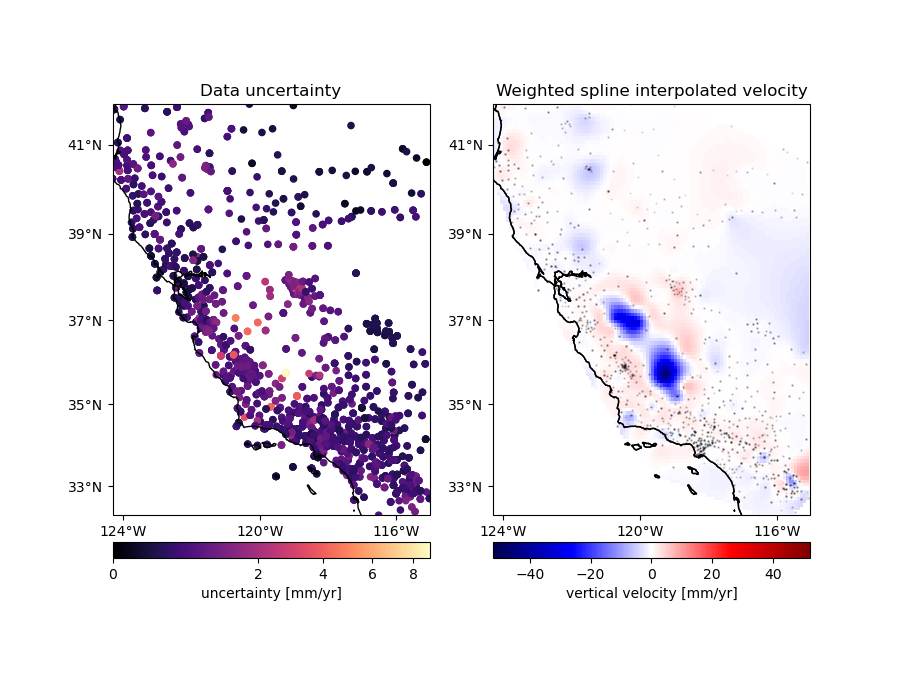# Gridding with splines and weights¶

An advantage of using the Green’s functions based `verde.Spline` over `verde.ScipyGridder` is that you can assign weights to the data to incorporate the data uncertainties or variance into the gridding. In this example, we’ll see how to combine `verde.BlockMean` to decimate the data and use weights based on the data uncertainty during gridding.Out:

```Chain(steps=[('mean', BlockMean(spacing=9250.0, uncertainty=True)),
('spline', Spline(damping=1e-10))])
/usr/share/miniconda3/envs/test/lib/python3.9/site-packages/verde/base/base_classes.py:359: FutureWarning: The default scoring will change from R² to negative root mean squared error (RMSE) in Verde 2.0.0. This may change model selection results slightly.
warnings.warn(

Score: 0.819
/usr/share/miniconda3/envs/test/lib/python3.9/site-packages/verde/base/base_classes.py:463: FutureWarning: The 'spacing', 'shape' and 'region' arguments will be removed in Verde v2.0.0. Please use the 'verde.grid_coordinates' function to define grid coordinates and pass them as the 'coordinates' argument.
warnings.warn(
```

```import cartopy.crs as ccrs
import matplotlib.pyplot as plt
import pyproj
from matplotlib.colors import PowerNorm

import verde as vd

# We'll test this on the California vertical GPS velocity data because it comes
# with the uncertainties
data = vd.datasets.fetch_california_gps()
coordinates = (data.longitude.values, data.latitude.values)

# Use a Mercator projection for our Cartesian gridder
projection = pyproj.Proj(proj="merc", lat_ts=data.latitude.mean())

# Now we can chain a block weighted mean and weighted spline together. We'll
# use uncertainty propagation to calculate the new weights from block mean
# because our data vary smoothly but have different uncertainties.
spacing = 5 / 60  # 5 arc-minutes
chain = vd.Chain(
[
("mean", vd.BlockMean(spacing=spacing * 111e3, uncertainty=True)),
("spline", vd.Spline(damping=1e-10)),
]
)
print(chain)

# Split the data into a training and testing set. We'll use the training set to
# grid the data and the testing set to validate our spline model. Weights need
# to 1/uncertainty**2 for the error propagation in BlockMean to work.
train, test = vd.train_test_split(
projection(*coordinates),
data.velocity_up,
weights=1 / data.std_up**2,
random_state=0,
)
# Fit the model on the training set
chain.fit(*train)
# And calculate an R^2 score coefficient on the testing set. The best possible
# score (perfect prediction) is 1. This can tell us how good our spline is at
# predicting data that was not in the input dataset.
score = chain.score(*test)
print("\nScore: {:.3f}".format(score))

# Create a grid of the vertical velocity and mask it to only show points close
# to the actual data.
region = vd.get_region(coordinates)
grid_full = chain.grid(
region=region,
spacing=spacing,
projection=projection,
dims=["latitude", "longitude"],
data_names=["velocity"],
)
(data.longitude, data.latitude), grid=grid_full, projection=projection
)

fig, axes = plt.subplots(
1, 2, figsize=(9, 7), subplot_kw=dict(projection=ccrs.Mercator())
)
crs = ccrs.PlateCarree()
# Plot the data uncertainties
ax = axes
ax.set_title("Data uncertainty")
# Plot the uncertainties in mm/yr and using a power law for the color scale to
# highlight the smaller values
pc = ax.scatter(
*coordinates,
c=data.std_up * 1000,
s=20,
cmap="magma",
transform=crs,
norm=PowerNorm(gamma=1 / 2)
)
cb = plt.colorbar(pc, ax=ax, orientation="horizontal", pad=0.05)
cb.set_label("uncertainty [mm/yr]")
vd.datasets.setup_california_gps_map(ax, region=region)
# Plot the gridded velocities
ax = axes
ax.set_title("Weighted spline interpolated velocity")
maxabs = vd.maxabs(data.velocity_up) * 1000
pc = (grid.velocity * 1000).plot.pcolormesh(
ax=ax,
cmap="seismic",
vmin=-maxabs,
vmax=maxabs,
transform=crs,